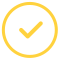effortlessly.

# Average Rate of Change Calculator – Math Cracker!

Are you at crossroads on what type of calculator to use for calculating your average rate of change? Well, you will find the average rate of change calculator a very useful tool. Find out how?

So, what is the average rate of change calculator? It is an easy to use online tool to calculate the average rate of change between two points over a given interval. With this online calculator, you can confidently say goodbye to any difficulties related to solving average rates of change.

Average Rate of Change Calculator

## How To Calculate the Average Rate of Change

Using the rate of change calculator, you will enter the A and B values, as well as the function f(x). The fast average rate of change calculator can handle any of the following operations and functions:

• Constants (e, `pi`, i imaginary unit)
• Operations (a+b, a-b)
• Functions (log a(x), ln(x))
• Trigonometric Functions (tan(x), tg(x), cot(x), ctg(x))
• Inverse Trigonometric Functions (asin(x), acsc(x))
• Hyperbolic Functions (sinh(x))
• Inverse Hyperbolic Functions such as asinh(x), arcsinh(x), sinh^-1(x))

All you need to do is to provide the necessary input, and this calculator will calculate rate of change for you.

### What is the Average Rate of Change Formula?

To find the rate of change for a function, you determine the change in the y-value and divide it by the x-value change for two points on the graph (ordered pairs).

Here is the average rate of change formula:

A = (f (C) - f (D)) / (C-D)

Where,

A = Average Rate of Change

C = C Value

D = D Value

An example would be:

Take a look at the equation 3x³ + 2x + 2, where A value equals 3 and B value equals 2. Determine the average rate of return for this function.

Solution:

f (a) = 3(3)³+ 3(3) + 2

= 81 + 6 + 2

= 89

f (b) = 3(2)³ + 2(2) + 2

= 24 + 4 + 2

= 30

Thus, average rate change = 89 - 30 / 3 - 2

= 59

The rate of change formula will therefore help you to solve the sum as fast possible.

### Why the Average Rate of Change Calculator is Easy to Use

There are several reasons why this calculator can be used by students of all levels painstakingly. They include:

• You do not need any special knowledge to use them: Once you have the formula and the values, you are good!
• The interface is user friendly: You can see where to enter the values to generate your answer.
• The average rate of change formula does not involve any complex expressions. Students can, therefore, use it with ease as compared to other formulae.

You can use the calculator by following the steps below:

1. Key in the values such as f(a), f(b), a value, and b value in the specified input field
2. Hit the “Calculate Average Rate of Change” button to get the results
3. You will get the average rate of change in a new window

### Calculator Is Not Enough? Try Our Online Math Homework Help

Our professional writers are ready to help in case the calculator answers are not enough. Try us today and enjoy cheap math homework help!

Let’s make your assignment go away.Awesome. Now you'll never miss out.